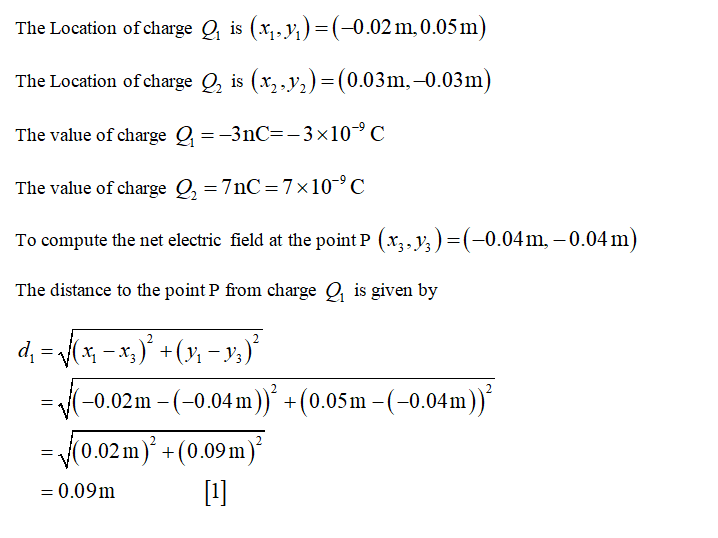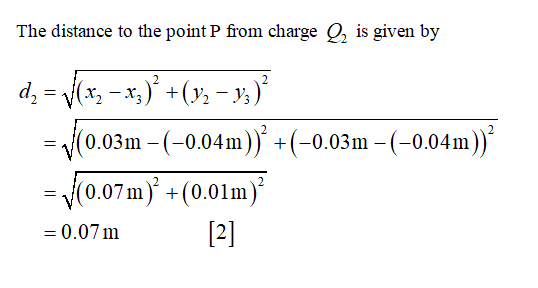Question
1 views

Two small charged objects are located as shown in the diagram. In the diagram each square represents 1e-2 m. Q1 = -3 nC and Q2 = 7 nC. You will calculate the net electric field at location  =  <−0.04, −0.04, 0>  m.

What is the electric field at  due to Q1?

What is the electric field at  due to Q2?

check_circle

Step 1Step 2...

### Want to see the full answer?

See Solution

#### Want to see this answer and more?

Solutions are written by subject experts who are available 24/7. Questions are typically answered within 1 hour.*

See Solution
*Response times may vary by subject and question.
Tagged in

### Physics